Historical Archive of the Bible Wheel Site

The Bible Wheel had been debunked by its author.
Read all about it: Debunking Myself: What A Long Strange Trip It's Been

Recent Blog ArticlesThus saith God the LORD, he that created the heavens, and stretched them out; he that spread forth the earth, and that which cometh out of it; he that giveth breath unto the people upon it, and spirit to them that walk therein:

Isaiah 42.5

The Number 36 = 6 x 6 is deeply related to the Work of Creation, which is based on the Number 6 in both the plain text and alphanumeric structure of Scripture. It is one less than the bifigurate Number 37 (the Heart of Wisdom), which can be represented as both a Hexagon and a Star:The extremely deep and profound integration of Genesis 1.1 with the Number 6 also emerges in these two identities:

 37 = 1 + 6 x 6 73 = 1 + 6 x 6 x 2

These numbers integrate with the Creation Holograph (recall Sum(N) denotes the Nth Triangular number):

 2701 = Sum(73) = 37 x 73 = 703 + 1998 = 703 + 3 x 666 = Sum(37) + 3 x Sum(36)

The last identity is based on the relation:

666 = Sum(36) = Sum(6 x 6)

The Number 1998 may also be written as twice the product of the first two elements of the Holographic Generating Set (A = 27, B = 37, C = 73)

1998 = 2 x 999 = 2 x 27 x 37

The image below shows the profound geometric meaning of the above numerical relations: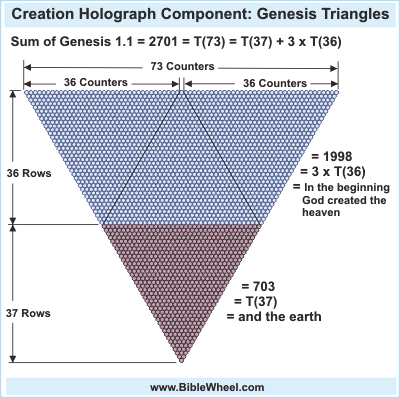This figure can be rearranged as three triangles, each containing 666 counters, surrounding the central triangle of 703 counters: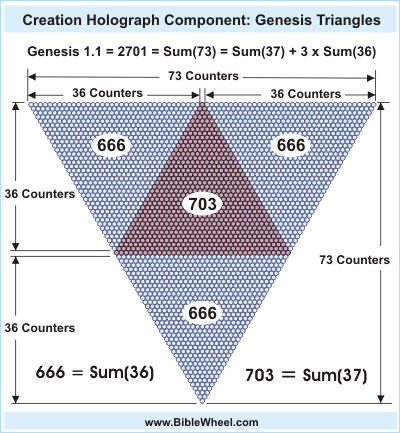The fact that 666 and 703 are consecutive triangular numbers means that their sum is the square of the larger triangle:

Sum(36) + Sum(37) = 666 + 703 = 37 x 37 = 1369 (Image of God, etc.)

Below are the primary identities associated with the Number 36. Note that the Holy of Holies (Davir) has the ordinal value of 36 and the standard value of 216 = 6 x 6 x 6, and that it contained the Ark of God = 343 = 7 x 7 x 7:

 The Number 36 God [Is 42.5]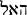HaEl Tabernacle, Tent [S# H0168]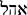Ohel Davir (Holy of Holies) (Ord) [1Ki 6.16]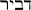by his power [Jer 10.12]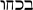B'Koachu Separate, Divide [S# H0914]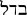Badal This is my beloved [Song 5.16]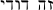Zah Dodi To/Lor him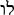Lu Lion (Ord) [Gen 49.9]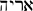Aryeh the Mighty (Ord) [Jer 32.18]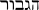HaGevurah

Factors: 6 x 6